# NCERT Solution Exercise 12.1 Heron’s Formula Class 9

Formula For the area of a triangle in different cases:

(i) When Base and Height are Given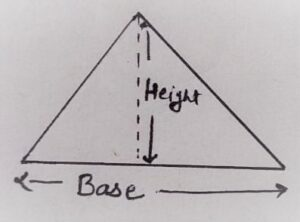Area of Triangle = 1/2 × ( Base × Height )

(ii) When all three sides are given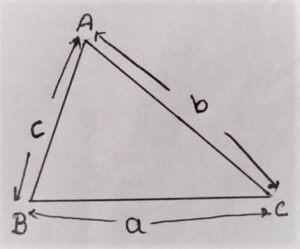S = (a+b+c)/2

By Heron’s Formula

Area of Triangle = √s(s-a)(s-b)(s-c)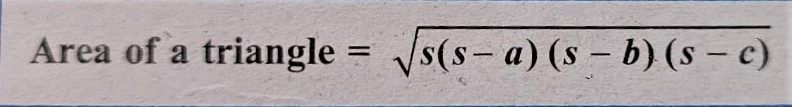Q1. A traffic signal board, including ‘SCHOOL AHEAD”, is an equilateral triangle with the side ‘a’. Find the area of the signal board, using Heron’s formula. If its perimeter is 180cm, what will be the area of the signal board?

Given:

Perimeter = 180 cm

Each side = a

To Find:

Area of signal board = ?

Solution: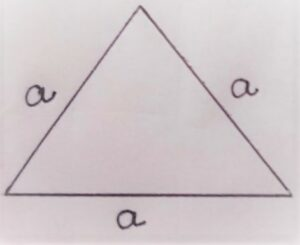sum of all three sides = perimeter of triangle

a + a + a = 180

⇒ 3a = 180

⇒ a = 180/3

⇒ a = 60

Area of Signal board = Area of Equilateral triangle

⇒ Area of Signal board = (√3/4 )a² [ This formula is derived by heron’s formula]

⇒ Area of Signal board = (√3/4 )60×60

⇒ Area of Signal board = √3 ×15 × 60

⇒ Area of Signal board = 900√3 cm²

Q2. The triangular side walls of a flyover have been used for advertisements. The sides of the walls are 122 m, 22m, and 120m(see Fig. 12.9). The advertisements yield an earning of Rs 5000 per m² per year. A company hired one of its walls for 3 months. How much rent did it pay?

Given: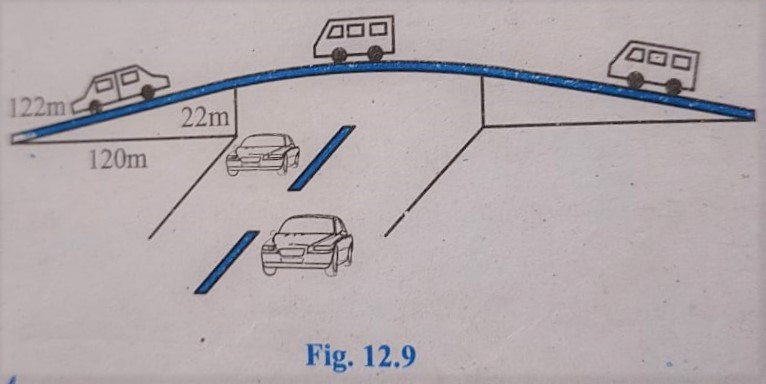a = 122 m

b = 120 m

c = 22 m

Per year earning 5000 per m²

The company hired one wall for 3 months =?

To Find:

Total Rent for 3 months for one wall =?

Solution:

s = (a +b +c)/2

⇒ s = (122+120+22)/2

⇒ s = 264/2

⇒ s = 132

(s-a) = 132-122 = 10

(s-b) = 132 – 120 = 12

(s-c) = 132 – 22 = 110

Area of one wall = Area of Triangle

⇒ Area of one wall = √s(s-a)(s-b)(s-c)

⇒ Area of one wall = √132×10×12×110

⇒ Area of one wall = √2×2×3×11×2×5×2×2×3×2×5×11

⇒ Area of one wall = √2×2×2×2×2×2×3×3×5×5×11×11

⇒ Area of one wall = 2×2×2×3×5×11

⇒ Area of one wall = 1320 m²

12 months rent = Rs 5000 per m²

1-month rent = Rs 5000/12 per m²

3 months rent = Rs 5000×3/12 = 1250 per m²

Total Length for 3 months for one wall = 1250×1320 = Rs 1650000

Q3. There is a slide in a park. One of its side walls has been painted in some colour with a message “KEEP THE PARK GREEN AND CLEAN”(see Fig. 12.10). If the sides of the wall are 15m, 11m, and 6m, find the area painted in colour.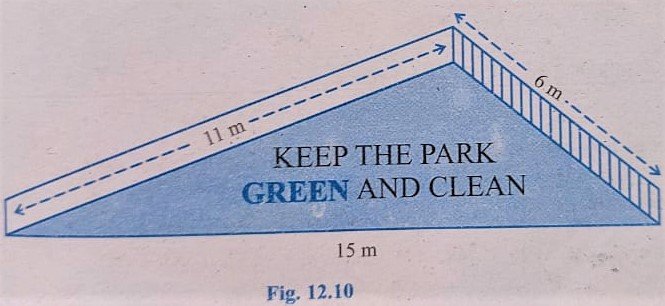Solution:

a = 15

b = 11

c = 6

s = (a +b +c)/2

⇒ s = (15+11+6)/2

⇒ s = 32/2

⇒ s = 16

(s-a) = 16-15 = 1

(s-b) = 16 – 11= 5

(s-c) = 16-6 = 10

By Heron’s Formula

Area of wall painted in colour = √s(s-a)(s-b)(s-c)

⇒ Area of wall painted in colour = √16(1)(5)(10)

⇒ Area of wall painted in colour = √16×5×5×2

⇒ Area of wall painted in colour = 4×5√2

⇒ Area of wall painted in colour = 20√2 m²

Q4. Find the area of a triangle two sides of which are 18 cm and 10 cm and the perimeter is 42 cm.

Given:

a = 18

b = 10

c = ?

To Find:

Area of Triangle =?

Solution:

s = perimeter/2 = 42/2 = 21

(s-a) = 21-18= 3

(s-b) = 21-10 = 11

(s-c) = 21- 14 = 7

Perimeter = 42

a +b + c = 42

⇒ 18 +10+c = 42

⇒ 28 +c = 42

⇒ c = 42 – 28

⇒ c = 14

By Heron’s Formula :

Area of Triangle = √s(s-a)(s-b)(s-c)

⇒ Area of Triangle = √21(3)(11)(7)

⇒ Area of Triangle = √3×7×3×11×7

⇒ Area of Triangle = 3×7√11

⇒ Area of Triangle = 21√11 cm²

Q5. Sides of a triangle are in the ratio of 12: 17: 25 and its perimeter is 540cm. Find its area.

Solution:

Let the sides are

a = 12x

b = 17x

c = 25x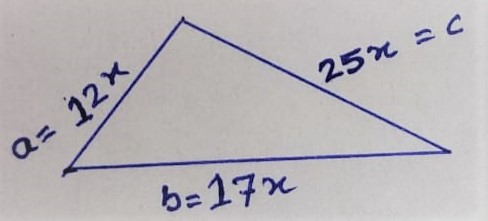Perimeter = 540 cm

the sum all three sides = Perimeter

12x + 17x + 25x = 540

54x = 540

x = 540/54

⇒ x = 10

a = 12×10 = 120 cm

b = 17×10 = 170 cm

c = 25×10 = 250 cm

S = Perimeter /2 = 540/2 = 270

By Heron’s Formula :

Area of Triangle = √s(s-a)(s-b)(s-c)

⇒ Area of Triangle = √270×120×170×250

⇒ Area of Triangle = √3×3×3×3×2×2×5×5×10×10×10×10×17

⇒ Area of Triangle = 3×3×2×5×10×10√17

⇒ Area of Triangle = 9000√17 cm²

Q6. An isosceles triangle has a perimeter of 30 cm and each of the equal sides is 12 cm. Find the area of the triangle.

Solution:

Let the  sides are a, b and chere a = c = 12

b = ?

Perimeter = 30

⇒ a + b + c = 30

⇒ 12+b+12 = 30

⇒ 24+b = 30

⇒ b = 30 – 24

⇒ b = 6

(s-a) = 15-12 = 3

(s-b) = 15-6 = 9

(s-c) = 15-12 = 3

By Heron’s Formula

Area of Triangle = √s(s-a)(s-b)(s-c)

⇒ Area of Triangle = √15(3)(9)(3)

⇒ Area of triangle = √3×5×3×3×3×3

⇒ Area of triangle = √3×3×3×3×3×5

⇒ Area of triangle = 3×3√3×5

⇒ Area of triangle = 9√15 cm²